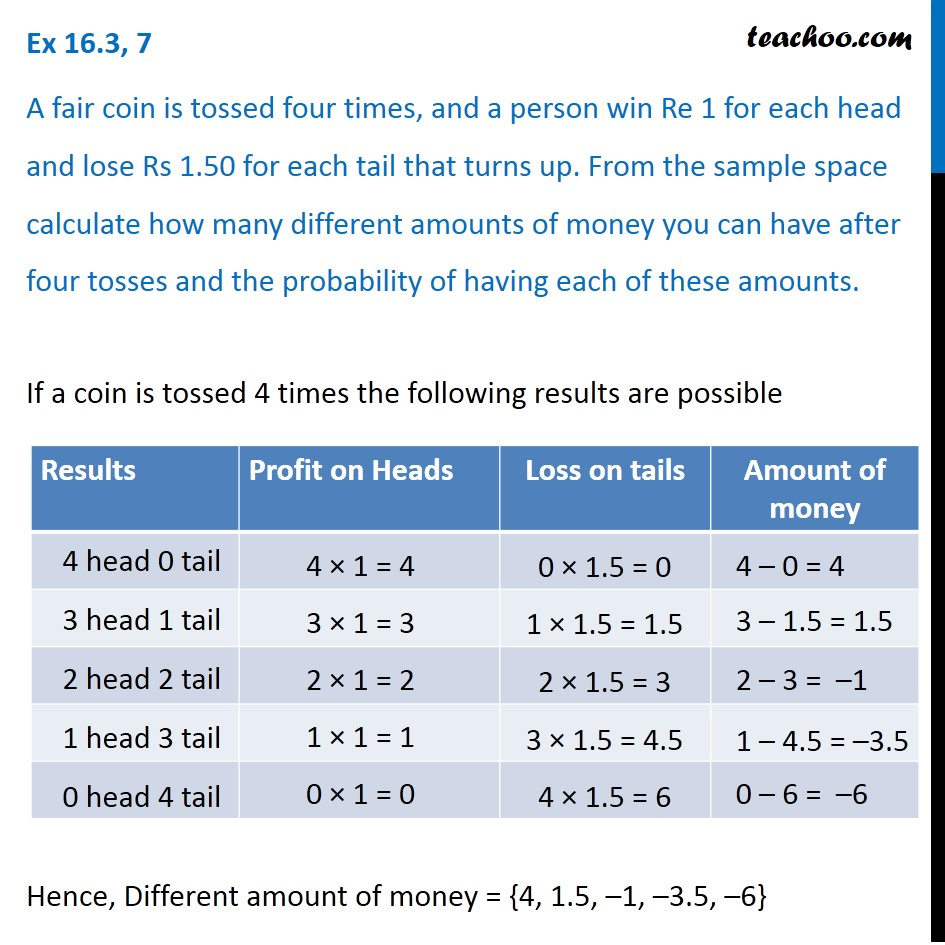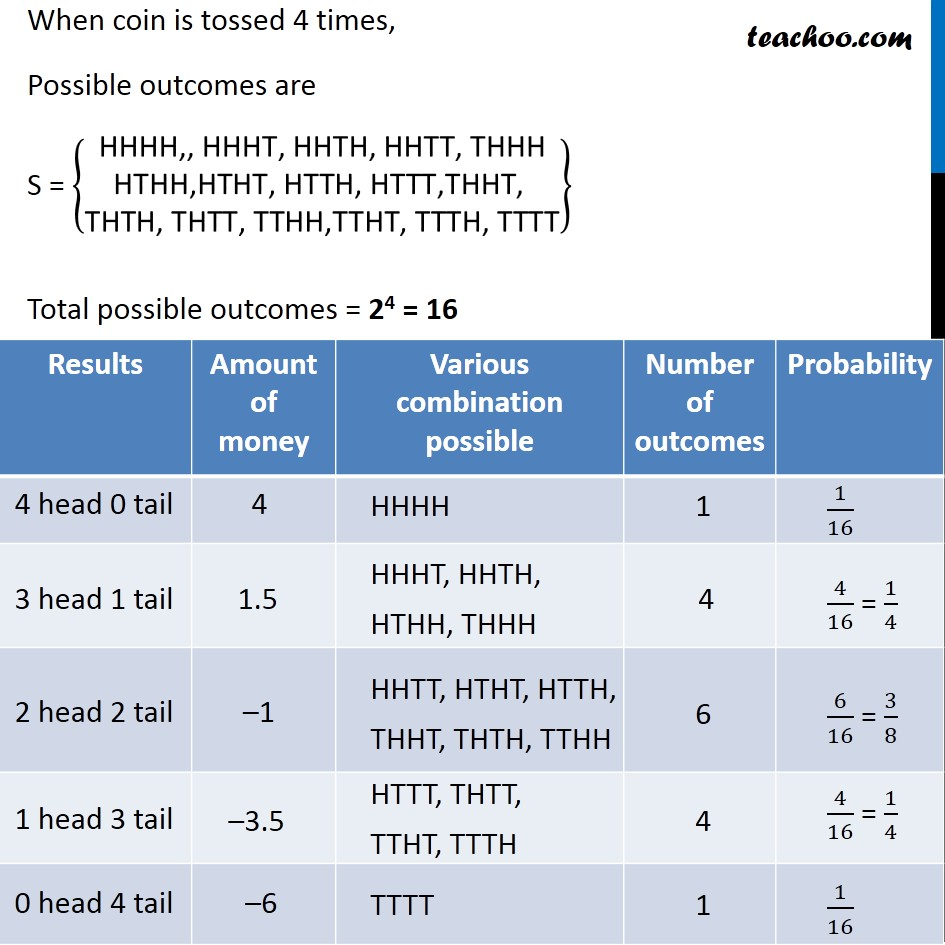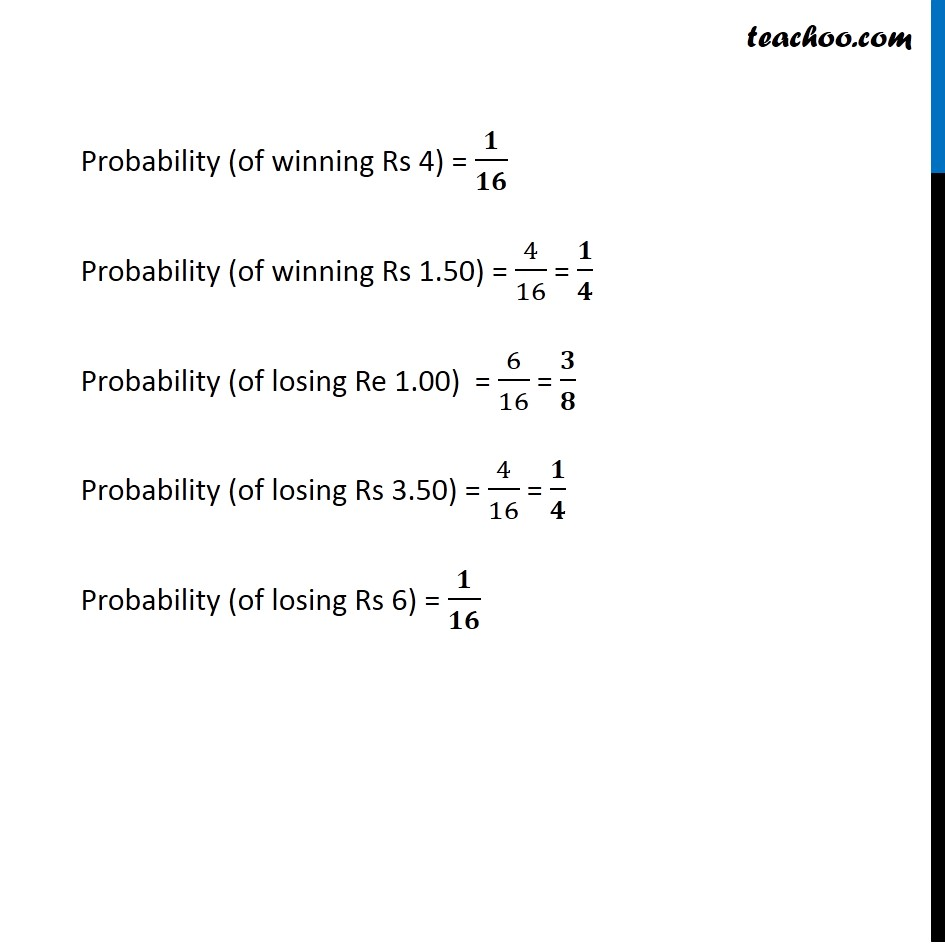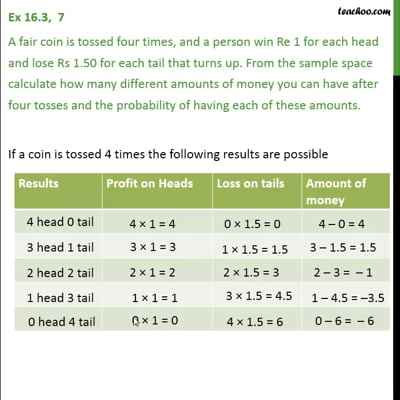Ex 16.3

Chapter 16 Class 11 Probability (Term 2)
Serial order wiseThis video is only available for Teachoo black users

### Transcript

Ex 16.3, 7 A fair coin is tossed four times, and a person win Re 1 for each head and lose Rs 1.50 for each tail that turns up. From the sample space calculate how many different amounts of money you can have after four tosses and the probability of having each of these amounts. If a coin is tossed 4 times the following results are possible Hence, Different amount of money = {4, 1.5, –1, –3.5, –6} When coin is tossed 4 times, Possible outcomes are S = {█("HHHH,, HHHT, HHTH, HHTT, THHH" @"HTHH,HTHT, HTTH, HTTT,THHT," @"THTH, THTT, TTHH,TTHT, TTTH, TTTT" )} Total possible outcomes = 24 = 16 Probability (of winning Rs 4) = 𝟏/𝟏𝟔 Probability (of winning Rs 1.50) = 4/16 = 𝟏/𝟒 Probability (of losing Re 1.00) = 6/16 = 𝟑/𝟖 Probability (of losing Rs 3.50) = 4/16 = 𝟏/𝟒 Probability (of losing Rs 6) = 𝟏/𝟏𝟔